# [ C++ ] 继承

面向对象编程的主要目的之一是提供可重用的代码，C++提供了一个方法来扩展和修改类。这种方法叫做类继承。它能够从已有的类派生出新的类，而派生类继承了原有类(称为基类)的特征，包括方法。

1.继承的概念和定义

1.1继承的概念

1.2 继承定义

1.2.1定义格式

1.2.2 继承关系和访问限定符

1.2.3 继承基类成员访问方式的变化

2.基类和派生类对象赋值转换

3. 继承中的作用域

4.派生类的默认成员函数

5. 继承与友元

6. 继承与静态成员

7. 菱形继承，菱形虚拟继承

7.1 菱形继承的问题

7.2 虚拟继承

8. 总结

# 1.继承的概念和定义

## 1.1继承的概念

从一个类派生出另一个类时，原始类称为基类，继承类称为派生类。为说明继承，首先需要一个基类。Person类中有_name，_age成员变量，有Print()成员方法。接下来我们将派生一个Student学生类

``````//父类、基类
class Person
{
public:
void Print()
{
cout << "name :" << _name << endl;
cout << "age :" << _age << endl;
}

//private:
//protected:
string _name = "Peter";
int _age = 18;
};``````

派生一个类：

派生类的声明方式，首先将Student类声明为从Person类派生而来：

``class Student : public Person``

冒号指出Student类的基类是Person类。public表示Person是一个公有基类，这被称为公有派生。派生类对象包含基类对象。使用公有派生，基类的公共成员将成为派生类的公有成员；基类的私有部分也将成为派生类的一部分，但只能通过基类的公有和保护方法访问。换句话说，Student类定义的对象s，可以使用Person类中的公有方法。

那么Student对象具有以下特征：

1.派生类对象存储了基类的数据成员（派生类继承了基类的实现）。

2.派生类对象可以使用基类的方法（派生类继承了基类的接口）。

派生类需要添加什么呢？

1. 派生类需要自己的构造函数。

2.派生类可以根据需要添加额外的数据成员和成员函数。

``````class Student : public Person
{
public:
void func()
{
Print();
}
protected:
int _stuid;//学号
};``````

我们可以看到Student类继承了Person类，并且是公有继承，并且Student类拥有自己额外的成员变量以及成员函数。## 1.2 继承定义

### 1.2.1定义格式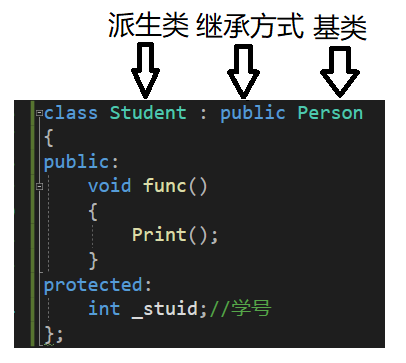### 1.2.2 继承关系和访问限定符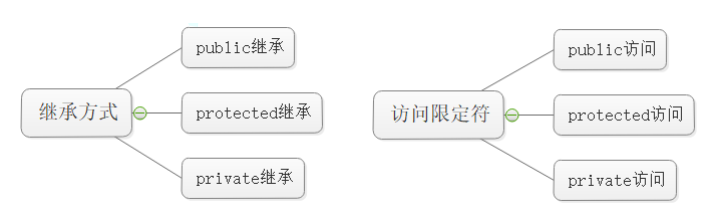### 1.2.3 继承基类成员访问方式的变化

 类成员 / 继承方式 public 继承 protected 继承 private 继承 基类的 public 成员 派生类的 public 成员 派生类的 protected 成员 派生类的 private 成员 基类的 protected 成员 派生类的 protected成员 派生类的 protected 成员 派生类的 private 成员 基类的 private 成 员 在派生类中 不可见 在派生类中 不可见 在派生类中 不可见

1. 基类 private 成员在派生类中无论以什么方式继承都是不可见的。这里的 不可见是指基类的私 有成员还是被继承到了派生类对象中，但是语法上限制派生类对象不管在类里面还是类外面 都不能去访问它
2. 基类 private 成员在派生类中是不能被访问，如果基类成员不想在类外直接被访问，但需要在 派生类中能访问，就定义为 protected 可以看出保护成员限定符是因继承才出现的

3. 实际上面的表格我们进行一下总结会发现，基类的私有成员在子类都是不可见。基类的其他成员在子类的访问方式 == Min(成员在基类的访问限定符，继承方式)public > protected

> private

4.使用关键字class时默认的继承方式是private，使用struct时默认的继承方式是public不过

5.在实际运用中一般使用都是public继承，几乎很少使用protetced/private继承，也不提倡

代码示例：

``````class Person
{
public:
void Print()
{
cout << "name:" << _name << endl;
cout << "age:" << _age << endl;
cout << "ID:" << _ID << endl;
}
public:
string _name = "张三";

protected:
int _age = 18;

private:
int _ID = 1;
};

class Student : public Person
{

};``````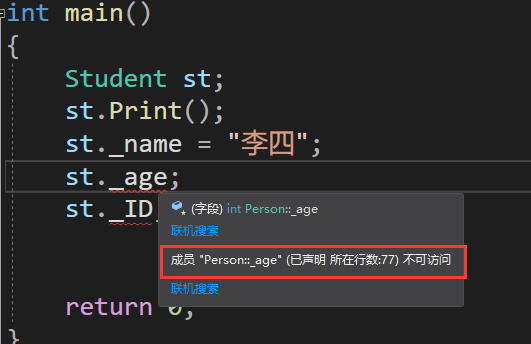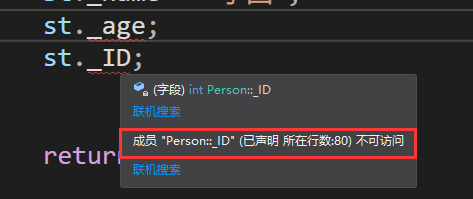# 2.基类和派生类对象赋值转换

• 派生类对象可以赋值类基类对象/基类的指针/基类的引用。这里有个形象的说法叫做切片或者切割。寓意把派生类中父类那部分切来赋值过去。
• 基类对象不能赋值给派生类对象。
• 基类的指针或者引用可以通过强制类型转换赋值给派生类的指针或者引用。但是必须是基类的指针是指向派生类对象时才是安全的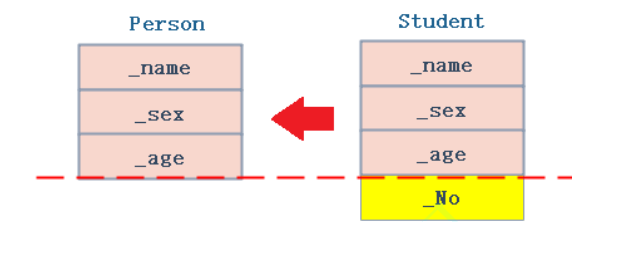``````class Person
{

//protected:
public:
string _name; // 姓名
string _sex;  // 性别
int	_age;	 // 年龄
};

class Student : public Person
{
public:
int _No; // 学号
};``````

1.子类对象给父类 对象/指针/引用 -- 语法天然支持，没有类型转换

``````    Person p;
Student s;
p = s;				//对象
Person& rp = s;		//引用
Person* ptrp = &s;	//指针``````

2.基类对象不能赋值给派生类对象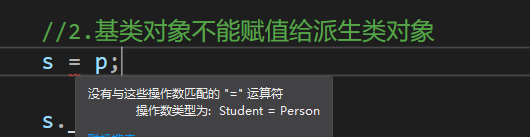3. 基类的指针可以通过强制类型转换赋值给派生类的指针

``````	ptrp = &s;
Student* ps1 = (Student*)ptrp;//这种情况下转换是可以的
ps1->_No = 10;

ptrp = &p;
Student* ps2 = (Student*)ptrp;//这种情况转换时虽然可以，但是会存在越界访问的问题
ps2->_No = 10;``````

# 3. 继承中的作用域

1. 在继承体系中 基类 派生类 都有 独立的作用域
2. 子类和父类中有同名成员，子类成员将屏蔽父类对同名成员的直接访问，这种情况叫隐藏，也叫重定义。 （在子类成员函数中，可以 使用 基类 :: 基类成员 显示访问
3. 需要注意的是如果是成员函数的隐藏，只需要函数名相同就构成隐藏。
4. 注意在实际中在 继承体系里 面最好 不要定义同名的成员
1. 成员变量导致隐藏关系
Student的_num和Person的_num构成隐藏关系，可以看出这样的代码虽然能跑，但是非常容易混淆，因此尽量避免重名。
``````class Person
{
protected:
string _name = "张三"; // 姓名
int _num = 111; 	    // 身份证号
};

class Student : public Person
{
public:
void Print()
{
cout << " 姓名:" << _name << endl;
cout << " 学号:" << _num << endl;//会使用自己的成员变量 999
cout << " 身份证号:" << Person::_num << endl; //要指定作用域
}
protected:
int _num = 999; // 学号
};
int main()
{
Student s;
s.Print();
return 0;
}``````

2. 成员方法导致隐藏关系

这段代码中b的fun()和A的fun()构成隐藏关系，b的fun()隐藏了A的fun()，若要使用A的fun()显示指定作用域即可访问到A的fun()。

``````class A
{
public:
void fun()
{
cout << "A::func()" << endl;
}
};
class B : public A
{
public:
void fun()
{
cout << "B::func()"<< endl;
}
};

int main()
{
B b;
b.fun();//b的fun()隐藏了A的fun()
b.A::fun();//指定作用域即可访问到A的func()
return 0;
};``````

# 4.派生类的默认成员函数

1. 派生类的构造函数必须调用基类的构造函数初始化基类的那一部分成员。如果基类没有默认
的构造函数，则必须在派生类构造函数的初始化列表阶段显示调用。
2. 派生类的拷贝构造函数必须调用基类的拷贝构造完成基类的拷贝初始化。
3. 派生类的 operator= 必须要调用基类的 operator= 完成基类的复制。
4. 派生类的析构函数会在被调用完成后自动调用基类的析构函数清理基类成员。因为这样才能 保证派生类对象先清理派生类成员再清理基类成员的顺序。
5. 派生类对象初始化先调用基类构造再调派生类构造。
6. 派生类对象析构清理先调用派生类析构再调基类的析构。
7. 因为后续一些场景析构函数需要构成重写， 重写的条件之一是函数名相同 那么编译器会对析构函数名进行特殊处理，处理成destrutor() ，所以父类析构函数不加 virtual 的情况下，子类析构函数和父类析构函数构成隐藏关系。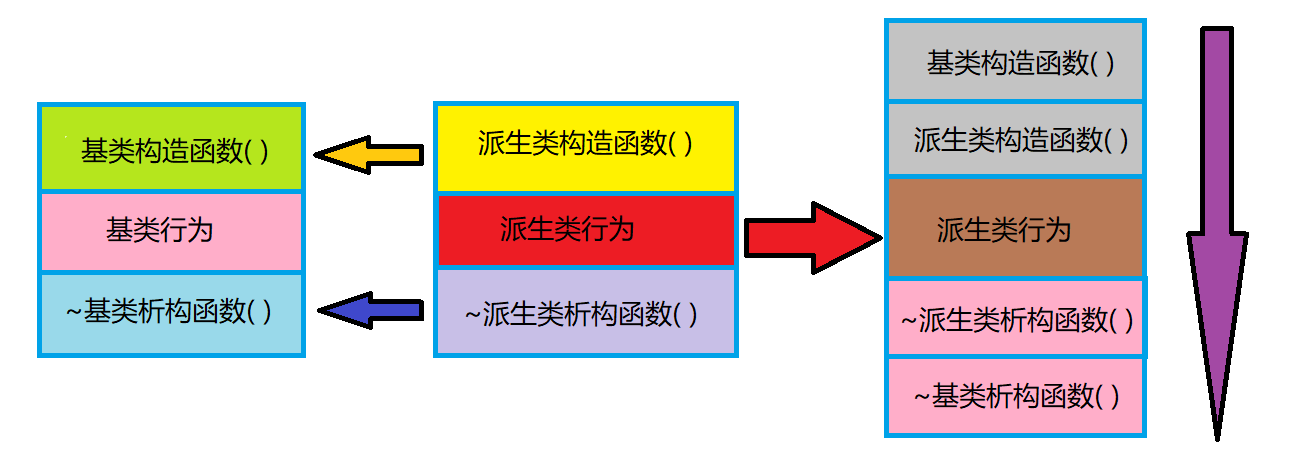``````class Person
{
public:
Person(const char* name)
:_name(name)
{
cout << "Person(const char* name)" << endl;
}
//拷贝构造
Person(const Person& p)
:_name(p._name)
{
cout << "Person(const Person& p)" << endl;
}

//赋值拷贝
Person& operator=(const Person& p)
{
cout << "Person& operator=(const Person& p)" << endl;
if (this != &p)
_name = p._name;

return *this;
}

~Person()
{
cout << "~Person()" << endl;
}

protected:
string _name;//姓名
};

class Student : public Person
{
public:
Student(const char* name = "", int num = 0)
:_num(num)
,Person(name)
{
cout << "Student(const char* name = "", int num = 0)" << endl;
}

//拷贝构造
Student(const Student& s)
:Person(s)
,_num(s._num)
{
cout << "Student(const Student& s)" << endl;
}

//赋值构造
// s1 = s3
Student& operator=(const Student& s)
{
if (this != &s)
{
Person::operator=(s);
_num = s._num;
}
cout << "Student& operator=(const Student& s)" << endl;

return *this;
}

//父类和子类析构函数构成隐藏关系
//原因：多态的需要，析构函数名同意会被处理成destructor()
//为了保证析构的顺序，先子后父
//子类析构函数完成后会自动调用父类析构函数,所以不需要我们显示调用
~Student()
{
//父类的析构函数我们不需要显示调用
//Person::~Person();
cout << "~Student()" << endl;
}
protected:
int _num; //学号

};``````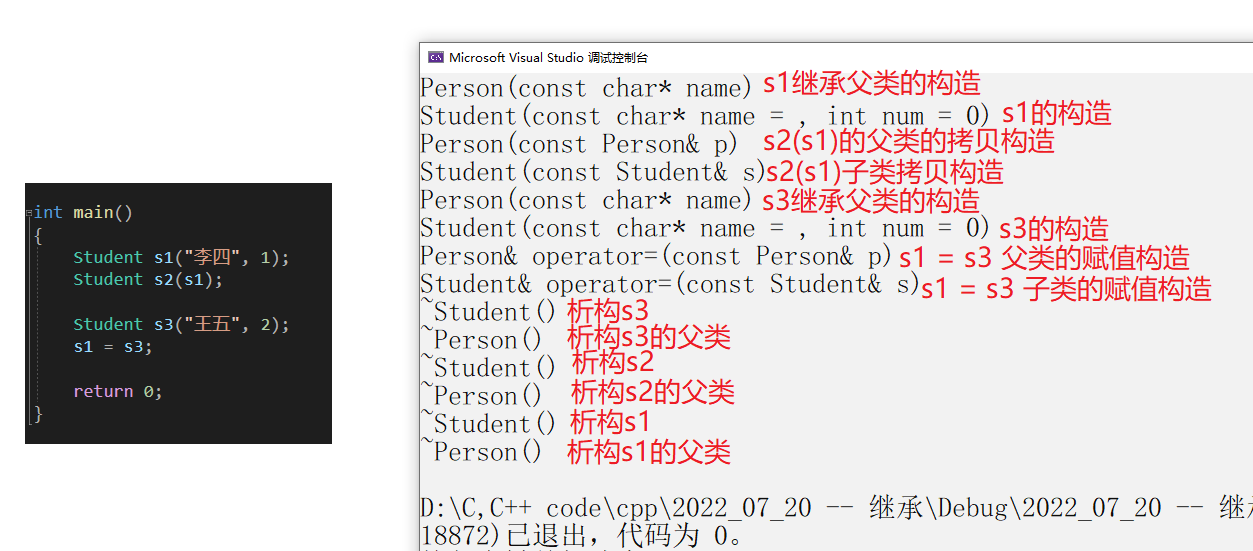# 5. 继承与友元

``````class Student;
class Person
{
public:
friend void Display(const Person& p, const Student& s);
protected:
string _name; // 姓名
};

class Student : public Person
{
friend void Display(const Person& p, const Student& s);
protected:
int _stuNum; // 学号
};

void Display(const Person& p, const Student& s)
{
cout << p._name << endl;
//要想访问到Student中的保护成员，要提供友元函数
cout << s._stuNum << endl;
}``````

# 6. 继承与静态成员

``````class Person
{
public:
Person() { ++_count; }
protected:
string _name; // 姓名
public:
static int _count; // 统计人的个数。
};

int Person::_count = 0;

class Student : public Person
{
protected:
int _stuNum; // 学号
};

{
protected:
string _seminarCourse; // 研究科目
};
//
int main()
{
Student s1;
Student s2;
Student s3;
Person s;

cout << " 人数 :" << Person::_count << endl;
cout << " 人数 :" << Student::_count << endl;
cout << " 人数 :" << s4._count << endl;

//同一个地址
cout << " 人数 :" << &Person::_count << endl;
cout << " 人数 :" << &Student::_count << endl;
cout << " 人数 :" << &s4._count << endl;

return 0;
}``````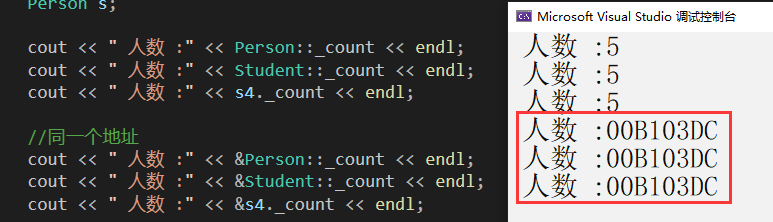# 7. 菱形继承，菱形虚拟继承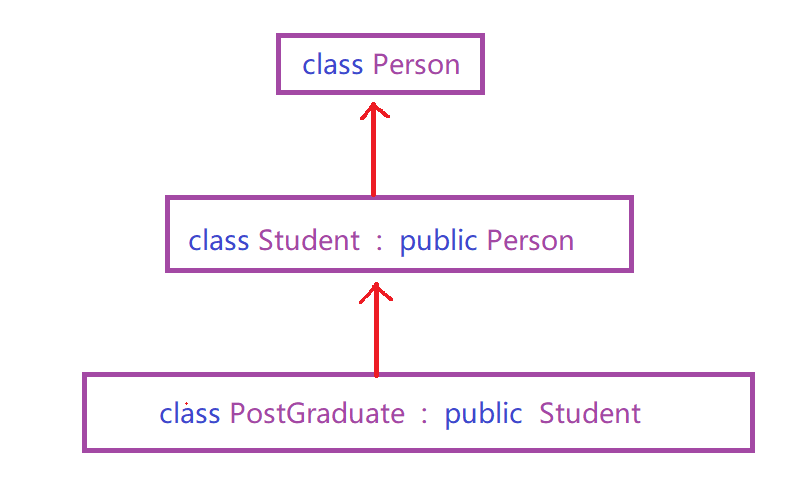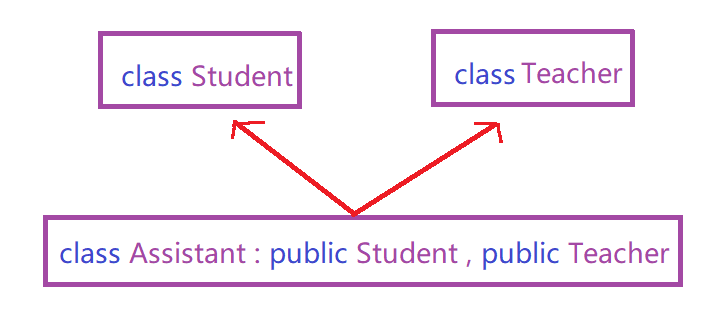菱形继承：菱形继承是多继承的一种特殊情况。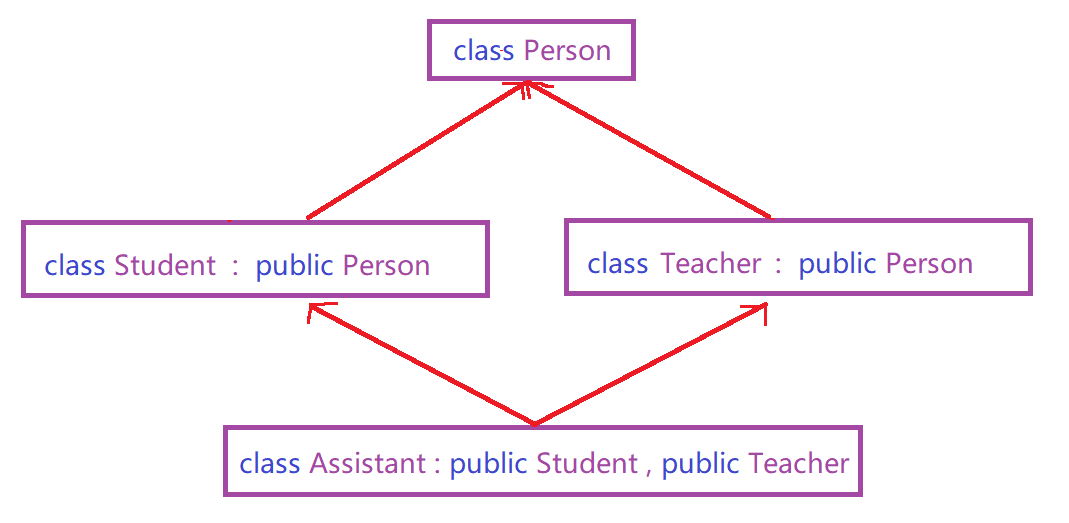## 7.1 菱形继承的问题

``````class Person
{
public:
string _name; // 姓名
int _a; //会有多份_a
};
class Student : virtual public Person
{
protected:
int _num; //学号
};
class Teacher : virtual public Person
{
protected:
int _id; // 职工编号
};
class Assistant : public Student, public Teacher
{
protected:
string _majorCourse; // 主修课程
};``````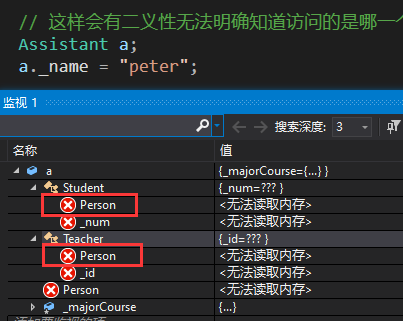## 7.2 虚拟继承

``````class A
{
public:
int _a;
//static int _a;
};

//int A::_a = 0;

//class B : public A
class B : virtual public A
{
public:
int _b;
};

//class C : public A
class C : virtual public A
{
public:
int _c;
};

class D : public B, public C
{
public:
int _d;
};``````

# 8. 总结

• 多继承 存在菱形继承，有了菱形继承就有菱形虚拟继承，底层实现就很复杂。所以一般不建议设计出多继承，一定不要设计出菱形继承。否则在复杂度及性能上都有问题
• 继承和组合         ​​​​​​​        ​​​​​​​        ​​​​​​​        ​​​​​​​        ​​​​​​​        ​​​​​​​        ​​​​​​​        ​​​​​​​        ​​​​​​​        ​​​​​​​        ​​​​​​​        ​​​​​​​        ​​​​​​​        ​​​​​​​        ​​​​​​​        1.    public继承是一种is-a的关系。也就是说每个派生类对象都是一个基类对象。​​​​​​​​​​​​​​                      2.    组合是一种 has-a 的关系。假设 B 组合了 A ，每个 B 对象中都有一个 A 对象。
• 在继承方式中，基类的 内部细节对子类可见 。继承一定程度破坏了基类的封装，基类的改变，对派生类有很大 的影响。派生类和基类间的依赖关系很强，耦合度高。
• 对象组合是类继承之外的另一种复用选择。对象组合要求被组合的对象具有良好定义的接口
因为对象的内部细节是不可见的。 组合类之间没有很强的依赖关系，耦合度低。优先使用对象组合有助于你保持每个类被 封装。
• 实际尽量多去用组合。组合的耦合度低，代码维护性好。不过继承也有用武之地的，有 些关系就适合继承那就用继承，另外要实现多态，也必须要继承。类之间的关系可以用 继承，可以用组合，就用组合。
``````// Car和BMW Car和Benz构成is-a的关系
class Car {
protected:
string _colour = "白色"; // 颜色
string _num = "陕ABIT00"; // 车牌号
};

class BMW : public Car {
public:
void Drive() { cout << "好开-操控" << endl; }
};

class Benz : public Car {
public:
void Drive() { cout << "好坐-舒适" << endl; }
};

// Tire和Car构成has-a的关系

class Tire {
protected:
string _brand = "Michelin";  // 品牌
size_t _size = 17;         // 尺寸
};

class Car {
protected:
string _colour = "白色"; // 颜色
string _num = "陕ABIT00"; // 车牌号
Tire _t; // 轮胎
};``````

(本篇完)